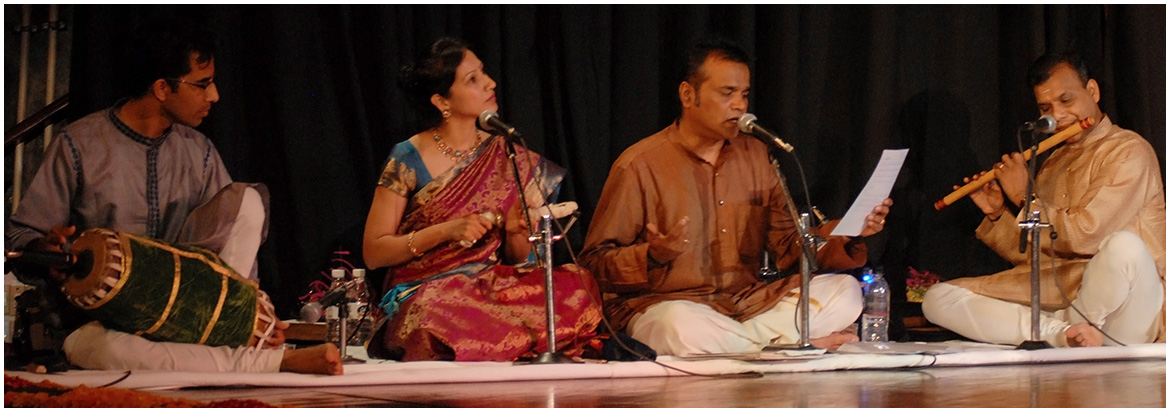# BlogCarnatic music is the life and blood of Bharatnatyam dance form and Taalams are the backbone of Carnatic music. Also known as Taalantainmah, derived from the Sanskrit word tala which literally means a clap, it is basically the rhythmic pattern or repetition of any composition. In Carnatic music this regular repeated rhythmic phrase is primarily rendered through the Mridangam, a percussionist instrument.

Each repeated cycle of a Talam is called an Aavartan. The first beat of the Talam is called Sam. A Talam is indicated visually by a series of rhythmic hand gestures and as these define a particular Talam, students have to master these.

Before we begin to convert any talam into a specific akshara kala, it is important to understand the 3 parts of a talam. These are:

1. Laghu symbolised as 1(can vary in number according to the jaati)i.e., 3(tisram), 4(chatusram), 5(khandam), 7(misram), and 9(sangeernam) aksharas.
2. Dhrutam symbolised as 0, it has 2 aksharas.
3. Anu dhrutam symbolised as U, it has 1 akshara.

Each talam comprises of a combination of one, two or all three of the above.

In order to create a tala of specified aksharas, we have to analyse all the talams and add the akshara kalas according to the jaatis appropriately.

Hence the talams which have 6 akshara kalas are as follows:

1. Rupakam-symbol = 01(dhrutam+laghu)=2+4(chatusra jaati)=6 akshara kalas
2. Jumpa-symbol = 1U0(laghu+anudhrutam+dhrutam)=3(tisram)+1+2=6 akshara kalas

The talams which have 7 akshara kalas are as follows:

1. Rupakam-symbol = 01(dhrutam+laghu)=2+5(khanada jaati)=7 akshara kalas
2. Triputa-symbol = 100(laghu+dhrutam +dhrutam)=3(tisram)+2+2=7 akshara kalas
3. Jumpa-symbol = 1U0(laghu+anudhrutam+dhrutam)=4(chatusram)+1+2=7 akshara kalas
4. Eka-symbol = 1(laghu)=7(misram)=7 akshara kalas

The talams which have 8 akshara kalas are as follows:

1. Matya-symbol = 101(laghu+dhrutam+laghu)=3(tisram)+2+3(tisram)=8 akshara kalas
2. Jumpa-symbol = 1U0(laghu+anu dhrutam +dhrutam)=5(khandam)+1+2=8 akshara kalas
3. Triputa-symbol = 100(laghu +dhrutam +dhrutam)=4(chatusram)+2+2=8 akshara kalas

The talams which have 9 akshara kalas are as follows:

1. Eka–symbol = 1(laghu)=9(sangeernam)=9 akshara kalas
2. Rupakam-symbol = 01(dhrutam +laghu)=2+7(misram)=9 akshara kalas
3. Triputa-symbol = 100(laghu+dhrutam+dhrutam)=5(khandam)+2+2=9 akshara kalas